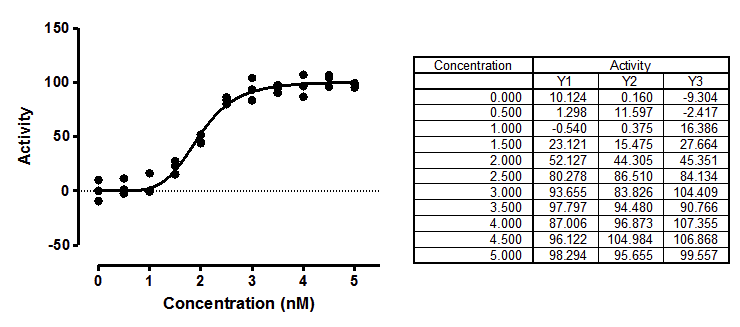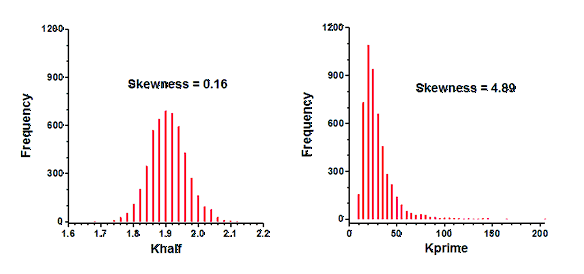## Please enable JavaScript to view this site.

 Reparameterizing an equation can help

Even though nonlinear regression, as its name implies, is designed to fit nonlinear models, some of the inferences actually assume that some aspects of the model are close to linear, so that the uncertainty about each parameter's value is symmetrical.

Reparameterizing an equation can make the uncertainty more symmetrical, making the SE easier to interpret and making the symmetrical asymptotic CI more helpful. Prism can compute an asymmetrical CI, and when you choose this approach it doesn't matter so much how you parametrize the equation.

## What is reparameterizing?

There are two forms of the model used to fit the sigmoidal enzyme kinetics data to a standard model:

Y=Vmax*X^h/(Khalf^h + X^h)

Y=Vmax*X^h/(Kprime + X^h)

The two equations are equivalent. They both fit Vmax (the maximum activity extrapolated to very high concentrations of substrate) and h (Hill slope, describing the steepness of the curve). But one model fits Khalf (the concentration needed to obtain a velocity half of maximal) and the other fits Kprime (a more abstract measure of substrate action).

Which model is best? The two are equivalent, with Kprime equal to Khalfh , so they will generate exactly the same curve.Since the sum-of-squares will be identical, as will the number of degrees of freedom, any comparison of this model with another will give identical results regardless of which form of this model you pick.

## Distribution of parameters are not always symmetrical

Simulations can determine how symmetrical a parameter's uncertainty is. I simulated sigmoidal enzyme kinetics using Vmax=100, h=5, Kprime=25 (so Khalf=5), and Gaussian scatter with a SD equal to 7.5. The X values matched those in the figure above, with triplicate Y values at each X. Prism can repeat such simulations easily. I repeated the simulations 5000 times, fit each curve to both forms of the models, and tabulated the best-fit values of Kprime and Khalf, and computed the skewness of each.Clearly the distribution of Khalf is quite symmetrical, and looks Gaussian. The skewness is close to zero, as expected for a symmetrical distribution. In contrast, the distribution of Kprime is quite skewed. Note that a few of the simulations had best-fit values of Kprime greater than 100. The skewness value (4.89) confirms what is obvious by inspection -- the distribution is far from symmetrical.

## Quantifying asymmetry with Hougaard's skewness

The results above were obtained by running numerous simulations. There is an easier way to figure out how symmetrical a parameter is. Prism can compute Hougaards skewness of each parameter, computed from the equation, the number of data points, the spacing of the X values, and the values of the parameters. For the simulated data set, Hougaard's skewness is 0.09 for Khalf and 1.83 for Kprime. A rule of thumb is to expect problems from asymmetry when the absolute value of the Hougaard's skewness is greater than 0.25, and big problems when the value is greater than 1.0. These values, which can be computed from one data set with no simulations, tells you that the symmetrical confidence intervals will be more accurate when you fit Khalf than when you fit Kprime.

Please note that while Prism 6 and 7 calculated Hougaard's skewness correctly for unweighted fits, they computed it incorrectly if you chose unequal weighting. This is fixed in Prism 8.

## Consequence of asymmetrical parameters

Ideally, a confidence interval is easy to interpret. A 95% CI has a 95% chance of including the true population value of the parameter, and a 5% chance of missing it.

When analyzing real data, we never know the value of the true parameter, so never know if the interval includes it or not. But when you simulate data, you know the true values of the parameters, so can quantify the coverage of the confidence intervals. I set up the same simulations mentioned above, fit each data set to both equations, and tabulated whether each confidence interval included the true parameter value or not. This table shows the fraction of  5,000 simulations where the asymptotic symmetrical confidence interval did not include the true parameter value (25 for Kprime, and 1.9037 for Khalf).

 "95% CI" "99% CI" Ideal 5.0% 1.0% Kprime 8.8% 4.8% Khalf 5.1% 1.0%

These results show that Khalf is well behaved, as expected given its symmetry (see above). The 95% confidence interval is expected to miss the true value in 5.0% of the simulations. In fact, it happened 5.1% of the time. Similarly, the 99% CI is expected to miss the true value in 1.0% of the simulations, which is exactly what happened. In contrast, Kprime is less well behaved. The intervals computed to be 95% confidence intervals were not wide enough so missed the true value in 8.8% of the simulations. The 99% intervals were similarly not wide enough so missed the true value in 4.8% of the simulations. Thus the  confidence intervals computed to be 99% intervals, actually turned out to be 95% intervals.

These simulations show the advantage of choosing the equation that fits Khalf, rather than the one that fits Kprime. Khalf has a symmetrical distribution so the confidence intervals computed from these fits can be interpreted at face value. In contrast, Kprime has an asymmetrical distribution and its confidence intervals cannot be interpreted at face value.

## Parameterization doesn't matter if you ask Prism to present the aymmetrical profile likelihood confidence intervals

If you choose asymmetrical profile likelihood confidence intervals, then it doesn't matter which form of the equation you choose. The coverage will be the same in both cases, and will be very close to 95% or 99%. With this choice, you can choose the form of the equation that matches text books and papers, or that fits the way you think. If you prefer to think graphically, choose the Khalf. If you think mechanistically, choose Kprime.